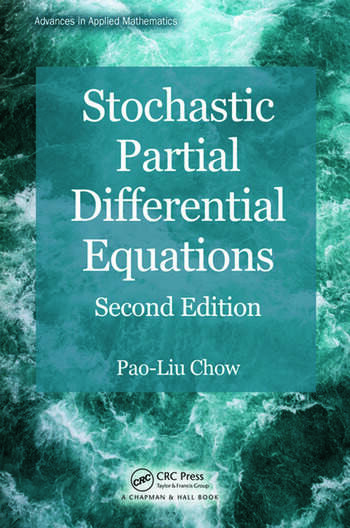# Stochastic Partial Differential Equations

## 2nd Edition

Pao-Liu Chow

Chapman and Hall/CRC
Published December 10, 2014
Reference - 334 Pages
ISBN 9781466579552 - CAT# K16851

For Instructors Request Inspection Copy

USD\$110.00

FREE Standard Shipping!

## Preview

### Summary

Explore Theory and Techniques to Solve Physical, Biological, and Financial Problems

Since the first edition was published, there has been a surge of interest in stochastic partial differential equations (PDEs) driven by the Lévy type of noise. Stochastic Partial Differential Equations, Second Edition incorporates these recent developments and improves the presentation of material.

New to the Second Edition

• Two sections on the Lévy type of stochastic integrals and the related stochastic differential equations in finite dimensions
• Discussions of Poisson random fields and related stochastic integrals, the solution of a stochastic heat equation with Poisson noise, and mild solutions to linear and nonlinear parabolic equations with Poisson noises
• Two sections on linear and semilinear wave equations driven by the Poisson type of noises
• Treatment of the Poisson stochastic integral in a Hilbert space and mild solutions of stochastic evolutions with Poisson noises
• Revised proofs and new theorems, such as explosive solutions of stochastic reaction diffusion equations
• Additional applications of stochastic PDEs to population biology and finance
• Updated section on parabolic equations and related elliptic problems in Gauss–Sobolev spaces

The book covers basic theory as well as computational and analytical techniques to solve physical, biological, and financial problems. It first presents classical concrete problems before proceeding to a unified theory of stochastic evolution equations and describing applications, such as turbulence in fluid dynamics, a spatial population growth model in a random environment, and a stochastic model in bond market theory. The author also explores the connection of stochastic PDEs to infinite-dimensional stochastic analysis.

#### Instructors

We provide complimentary e-inspection copies of primary textbooks to instructors considering our books for course adoption.